# Precision and representation issues¶

Most of the time, using mpmath is simply a matter of setting the desired precision and entering a formula. For verification purposes, a quite (but not always!) reliable technique is to calculate the same thing a second time at a higher precision and verifying that the results agree.

To perform more advanced calculations, it is important to have some understanding of how mpmath works internally and what the possible sources of error are. This section gives an overview of arbitrary-precision binary floating-point arithmetic and some concepts from numerical analysis.

The following concepts are important to understand:

• The main sources of numerical errors are rounding and cancellation, which are due to the use of finite-precision arithmetic, and truncation or approximation errors, which are due to approximating infinite sequences or continuous functions by a finite number of samples.
• Errors propagate between calculations. A small error in the input may result in a large error in the output.
• Most numerical algorithms for complex problems (e.g. integrals, derivatives) give wrong answers for sufficiently ill-behaved input. Sometimes virtually the only way to get a wrong answer is to design some very contrived input, but at other times the chance of accidentally obtaining a wrong result even for reasonable-looking input is quite high.
• Like any complex numerical software, mpmath has implementation bugs. You should be reasonably suspicious about any results computed by mpmath, even those it claims to be able to compute correctly! If possible, verify results analytically, try different algorithms, and cross-compare with other software.

## Precision, error and tolerance¶

The following terms are common in this documentation:

• Precision (or working precision) is the precision at which floating-point arithmetic operations are performed.
• Error is the difference between a computed approximation and the exact result.
• Accuracy is the inverse of error.
• Tolerance is the maximum error (or minimum accuracy) desired in a result.

Error and accuracy can be measured either directly, or logarithmically in bits or digits. Specifically, if a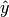is an approximation for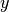, then

• (Direct) absolute error =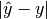• (Direct) relative error =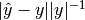• (Direct) absolute accuracy =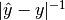• (Direct) relative accuracy =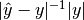• (Logarithmic) absolute error =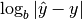• (Logarithmic) relative error =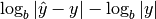• (Logarithmic) absolute accuracy =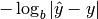• (Logarithmic) relative accuracy =where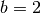and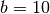for bits and digits respectively. Note that:

• The logarithmic error roughly equals the position of the first incorrect bit or digit
• The logarithmic accuracy roughly equals the number of correct bits or digits in the result

These definitions also hold for complex numbers, using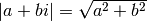.

Full accuracy means that the accuracy of a result at least equals prec-1, i.e. it is correct except possibly for the last bit.

## Representation of numbers¶

Mpmath uses binary arithmetic. A binary floating-point number is a number of the form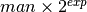where both man (the mantissa) and exp (the exponent) are integers. Some examples of floating-point numbers are given in the following table.

Number Mantissa Exponent
3 3 0
10 5 1
-16 -1 4
1.25 5 -2

The representation as defined so far is not unique; one can always multiply the mantissa by 2 and subtract 1 from the exponent with no change in the numerical value. In mpmath, numbers are always normalized so that man is an odd number, with the exception of zero which is always taken to have man = exp = 0. With these conventions, every representable number has a unique representation. (Mpmath does not currently distinguish between positive and negative zero.)

Simple mathematical operations are now easy to define. Due to uniqueness, equality testing of two numbers simply amounts to separately checking equality of the mantissas and the exponents. Multiplication of nonzero numbers is straightforward: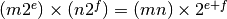. Addition is a bit more involved: we first need to multiply the mantissa of one of the operands by a suitable power of 2 to obtain equal exponents.

More technically, mpmath represents a floating-point number as a 4-tuple (sign, man, exp, bc) where sign is 0 or 1 (indicating positive vs negative) and the mantissa is nonnegative; bc (bitcount) is the size of the absolute value of the mantissa as measured in bits. Though redundant, keeping a separate sign field and explicitly keeping track of the bitcount significantly speeds up arithmetic (the bitcount, especially, is frequently needed but slow to compute from scratch due to the lack of a Python built-in function for the purpose).

Contrary to popular belief, floating-point numbers do not come with an inherent “small uncertainty”, although floating-point arithmetic generally is inexact. Every binary floating-point number is an exact rational number. With arbitrary-precision integers used for the mantissa and exponent, floating-point numbers can be added, subtracted and multiplied exactly. In particular, integers and integer multiples of 1/2, 1/4, 1/8, 1/16, etc. can be represented, added and multiplied exactly in binary floating-point arithmetic.

Floating-point arithmetic is generally approximate because the size of the mantissa must be limited for efficiency reasons. The maximum allowed width (bitcount) of the mantissa is called the precision or prec for short. Sums and products of floating-point numbers are exact as long as the absolute value of the mantissa is smaller than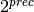. As soon as the mantissa becomes larger than this, it is truncated to contain at most prec bits (the exponent is incremented accordingly to preserve the magnitude of the number), and this operation introduces a rounding error. Division is also generally inexact; although we can add and multiply exactly by setting the precision high enough, no precision is high enough to represent for example 1/3 exactly (the same obviously applies for roots, trigonometric functions, etc).

The special numbers +inf, -inf and nan are represented internally by a zero mantissa and a nonzero exponent.

Mpmath uses arbitrary precision integers for both the mantissa and the exponent, so numbers can be as large in magnitude as permitted by the computer’s memory. Some care may be necessary when working with extremely large numbers. Although standard arithmetic operators are safe, it is for example futile to attempt to compute the exponential function of of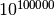. Mpmath does not complain when asked to perform such a calculation, but instead chugs away on the problem to the best of its ability, assuming that computer resources are infinite. In the worst case, this will be slow and allocate a huge amount of memory; if entirely impossible Python will at some point raise OverflowError: long int too large to convert to int.

For further details on how the arithmetic is implemented, refer to the mpmath source code. The basic arithmetic operations are found in the libmp directory; many functions there are commented extensively.

## Decimal issues¶

Mpmath uses binary arithmetic internally, while most interaction with the user is done via the decimal number system. Translating between binary and decimal numbers is a somewhat subtle matter; many Python novices run into the following “bug” (addressed in the General Python FAQ):

>>> 1.2 - 1.0
0.19999999999999996


Decimal fractions fall into the category of numbers that generally cannot be represented exactly in binary floating-point form. For example, none of the numbers 0.1, 0.01, 0.001 has an exact representation as a binary floating-point number. Although mpmath can approximate decimal fractions with any accuracy, it does not solve this problem for all uses; users who need exact decimal fractions should look at the decimal module in Python’s standard library (or perhaps use fractions, which are much faster).

With prec bits of precision, an arbitrary number can be approximated relatively to within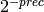, or within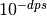for dps decimal digits. The equivalent values for prec and dps are therefore related proportionally via the factor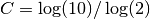, or roughly 3.32. For example, the standard (binary) precision in mpmath is 53 bits, which corresponds to a decimal precision of 15.95 digits.

More precisely, mpmath uses the following formulas to translate between prec and dps:

dps(prec) = max(1, int(round(int(prec) / C - 1)))

prec(dps) = max(1, int(round((int(dps) + 1) * C)))


Note that the dps is set 1 decimal digit lower than the corresponding binary precision. This is done to hide minor rounding errors and artifacts resulting from binary-decimal conversion. As a result, mpmath interprets 53 bits as giving 15 digits of decimal precision, not 16.

The dps value controls the number of digits to display when printing numbers with str(), while the decimal precision used by repr() is set two or three digits higher. For example, with 15 dps we have:

>>> from mpmath import *
>>> mp.dps = 15
>>> str(pi)
'3.14159265358979'
>>> repr(+pi)
"mpf('3.1415926535897931')"


The extra digits in the output from repr ensure that x == eval(repr(x)) holds, i.e. that numbers can be converted to strings and back losslessly.

It should be noted that precision and accuracy do not always correlate when translating between binary and decimal. As a simple example, the number 0.1 has a decimal precision of 1 digit but is an infinitely accurate representation of 1/10. Conversely, the number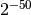has a binary representation with 1 bit of precision that is infinitely accurate; the same number can actually be represented exactly as a decimal, but doing so requires 35 significant digits:

0.00000000000000088817841970012523233890533447265625


All binary floating-point numbers can be represented exactly as decimals (possibly requiring many digits), but the converse is false.

## Correctness guarantees¶

Basic arithmetic operations (with the mp context) are always performed with correct rounding. Results that can be represented exactly are guranteed to be exact, and results from single inexact operations are guaranteed to be the best possible rounded values. For higher-level operations, mpmath does not generally guarantee correct rounding. In general, mpmath only guarantees that it will use at least the user-set precision to perform a given calculation. The user may have to manually set the working precision higher than the desired accuracy for the result, possibly much higher.

Functions for evaluation of transcendental functions, linear algebra operations, numerical integration, etc., usually automatically increase the working precision and use a stricter tolerance to give a correctly rounded result with high probability: for example, at 50 bits the temporary precision might be set to 70 bits and the tolerance might be set to 60 bits. It can often be assumed that such functions return values that have full accuracy, given inputs that are exact (or sufficiently precise approximations of exact values), but the user must exercise judgement about whether to trust mpmath.

The level of rigor in mpmath covers the entire spectrum from “always correct by design” through “nearly always correct” and “handling the most common errors” to “just computing blindly and hoping for the best”. Of course, a long-term development goal is to successively increase the rigor where possible. The following list might give an idea of the current state.

Operations that are correctly rounded:

• Addition, subtraction and multiplication of real and complex numbers.
• Division and square roots of real numbers.
• Powers of real numbers, assuming sufficiently small integer exponents (huge powers are rounded in the right direction, but possibly farther than necessary).
• Conversion from decimal to binary, for reasonably sized numbers (roughly between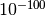and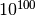).
• Typically, transcendental functions for exact input-output pairs.

Operations that should be fully accurate (however, the current implementation may be based on a heuristic error analysis):

• Radix conversion (large or small numbers).
• Mathematical constants like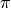.
• Both real and imaginary parts of exp, cos, sin, cosh, sinh, log.
• Other elementary functions (the largest of the real and imaginary part).
• The gamma and log-gamma functions (the largest of the real and the imaginary part; both, when close to real axis).
• Some functions based on hypergeometric series (the largest of the real and imaginary part).

Correctness of root-finding, numerical integration, etc. largely depends on the well-behavedness of the input functions. Specific limitations are sometimes noted in the respective sections of the documentation.

## Double precision emulation¶

On most systems, Python’s float type represents an IEEE 754 double precision number, with a precision of 53 bits and rounding-to-nearest. With default precision (mp.prec = 53), the mpmath mpf type roughly emulates the behavior of the float type. Sources of incompatibility include the following:

• In hardware floating-point arithmetic, the size of the exponent is restricted to a fixed range: regular Python floats have a range between roughly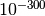and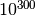. Mpmath does not emulate overflow or underflow when exponents fall outside this range.
• On some systems, Python uses 80-bit (extended double) registers for floating-point operations. Due to double rounding, this makes the float type less accurate. This problem is only known to occur with Python versions compiled with GCC on 32-bit systems.
• Machine floats very close to the exponent limit round subnormally, meaning that they lose accuracy (Python may raise an exception instead of rounding a float subnormally).
• Mpmath is able to produce more accurate results for transcendental functions.

## Further reading¶

There are many excellent textbooks on numerical analysis and floating-point arithmetic. Some good web resources are:

### Table Of Contents

#### Previous topic

Number identification

References